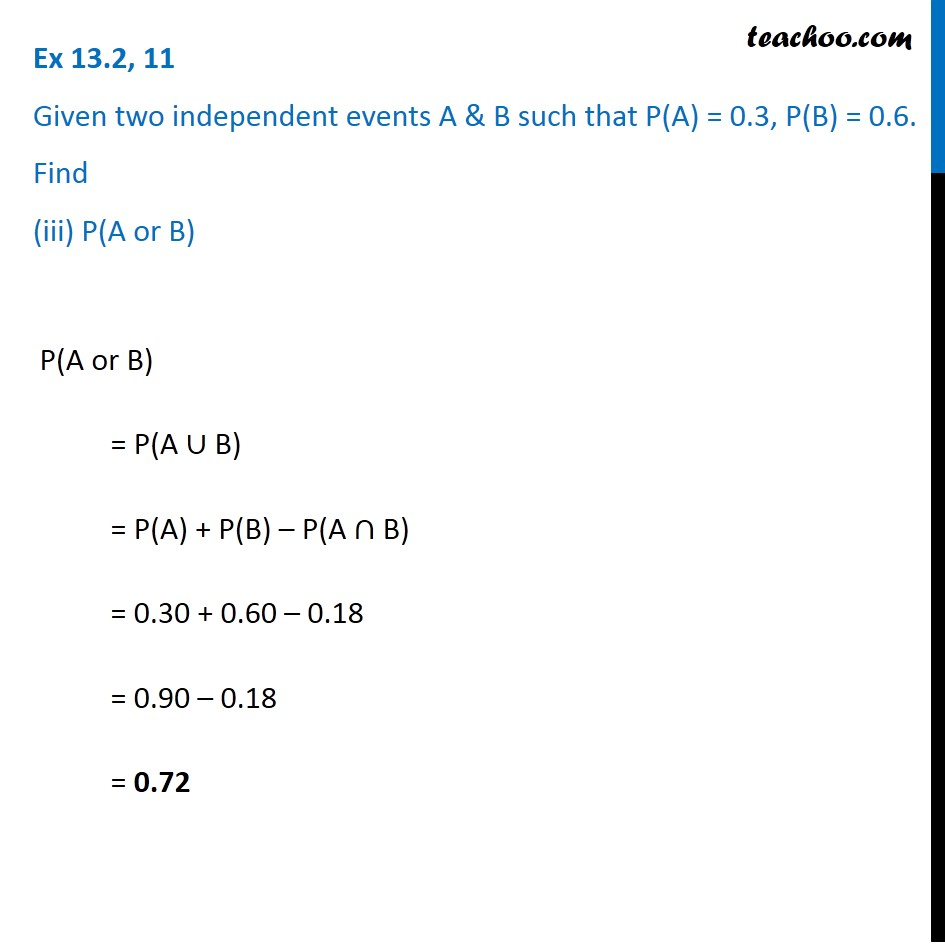Ex 13.2

Chapter 13 Class 12 Probability
Serial order wiseIntroducing your new favourite teacher - Teachoo Black, at only ₹83 per month

### Transcript

Ex 13.2, 11 Given two independent events A & B such that P(A) = 0.3, P(B) = 0.6. Find (iii) P(A or B)P(A or B) = P(A ∪ B) = P(A) + P(B) – P(A ∩ B) = 0.30 + 0.60 – 0.18 = 0.90 – 0.18 = 0.72